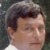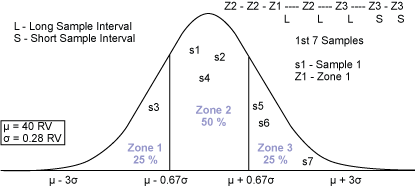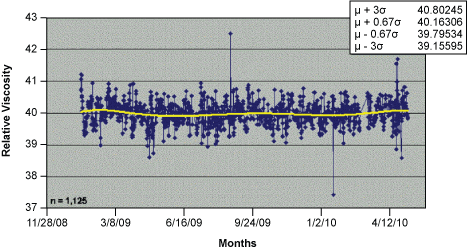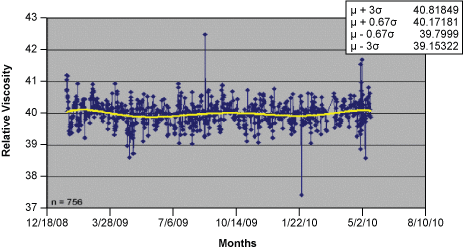# Reducing Sampling Costs: Implementing a Variable Sampling Interval Strategy

ByRichard Miller

Most manufacturing processes are controlled by sampling a product at some regular interval. Often, when a process is running normally, this interval is once every shift. It is not too surprising that in today’s economic climate, where cutting cost is of paramount importance, reducing sampling to save money is inviting, especially at large manufacturing facilities, where lots of measurements are routinely made. Of course, anytime an organization reduces sampling, the risk increases for making off-grade material over the longer, untested interval. The trick, then, is to reduce sampling in such a way as to minimize this risk – and that is what a variable sampling interval (VSI) strategy accomplishes.

### How VSI Works

Variable sampling interval theory, has been outlined in a number of journal articles over the past few decades (see “Resources” at the end of this article). However, it has been slow to be adopted industrially, largely because the imposition of two or more sampling intervals on a process adds complication. But VSI does offer cost-reduction potential and thus is becoming a subject for more serious consideration. In a nutshell, when a process is running at low risk, practitioners using VSI will decrease sampling frequency to, say, every other shift. In more risky circumstances, the normal sampling frequency is used. To apply the VSI approach in a manufacturing setting, a practitioner simply adds a few more lines (+/- 0.67σ) to the control chart and adopts switching rules so they know when to use which interval.

### Nylon Polymer Example Applying VSI

Consider a manufacturer that makes nylon polymer which must meet relative viscosity (RV) specifications (40 +/-1 RV) for purposes of controlling the polymer’s molecular weight. Day after day, it produces polymer exhibiting a normal distribution of RV values (Figure 1). To implement a simple VSI strategy, the practitioner divides that distribution into three zones:

• Zone 1 (between µ – 3σ and µ – 0.67σ)
• Zone 2 (between µ – 0.67σ and µ + 0.67σ)
• Zone 3 (between µ + 0.67σ and µ + 3.0σ)

In a perfectly normal distribution, the day-to-day sample RVs can then be expected to fall into these three zones 25, 50 and 25 percent of the time, respectively.Figure 1: Distribution of Nylon Polymer’s Relative Viscosity

To implement this VSI strategy, a practitioner puts the additional +/- 0.67σ lines on the Shewhart chart and institutes two simple rules:

• If two of three samples fall in Zones 1 or 3, sample the short interval next.
• If two of three samples fall in Zone 2, sample the long interval next.

The locations of the first seven samples (S1 to S7) are shown in Figure 1, along with their respective sampling periods: three shorts, as the data stream began, followed by three longs and two shorts; the eighth sample would be taken at the short interval because two of the three immediately preceding samples fell within Zone 3. Note: For purposes here, the short interval is the next shift, while the long interval would be the one after (i.e., a shift is skipped).

Table 1 characterizes the 10 possible states and their associated probabilities for any grouping of three consecutive data points.

Table 1: Probability Matrix for Three-point VSI Strategy

 Three-sample States Zonal Probabilities for Last Three Data Points Sampling Period Zone 1 Zone 2 Zone 3 Unshifted +1 sigma process shift 1 111 (0.24865)3 0.015373 (0.0474)3 0.00011 Short 2 111 (0.50)3 0.125 (0.3232)3 0.03376 Long 3 111 (0.24865)3 0.015373 (0.6066)3 0.22321 Short 4 11 1 3(0.25)2(0.5) 0.09274 3(0.0474)2(0.3232) 0.00218 Short 5 11 1 3(0.25)2(0.25) 0.04612 3(0.0474)2(0.6066) 0.00409 Short 6 1 11 3(0.25)2(0.5)2 0.186488 3(0.0474)2(0.3232)2 0.01485 Long 7 11 1 3(0.5)2(0.25) 0.186488 3(0.3232)2(0.6066) 0.19009 Long 8 1 11 3(0.25)(0.25)2 0.04612 3(0.0474)(0.6066)2 0.05232 Short 9 1 11 3(0.5)(0.25)2 0.09274 3(0.3232)(0.6066)2 0.35678 Short 10 1 1 1 6(0.25)2(0.5) 0.18548 6(0.0474)(0.3232)(0.6066) 0.05576 Long Zone 1: 25%, Zone 2: 50%, Zone 3: 25% Total prob: 0.99192Long prob: 0.683455Short prob: 0.3084663-sigma violation prob: 0.00808or 1 chance in: 123.7907 Switching rules: 2/3 Zone 1 = sample short, 2/3 Zone 2 = sample long, 2/3 Zone 3 = sample short 0.9331 0.294465 0.638682 0.06685 14.95834

For example, a practitioner could expect the probability of all three points falling within either Zone 1 or Zone 3 to be 1.54 percent, and 12.5 percent for Zone 2. The three highest-probability states, each occurring 18.65 percent of the time, either have two points in Zone 2 and one in either Zone 1 or Zone 3, or one point in each of the three zones. All three would then require that the next sampling interval be long. Note: The probabilities for these 10 states add up to 0.99192 because there is a 0.00808 probability that one or more of the three points would fall beyond the 3σ limits and trigger a special-cause event – in this case a false signal.

The far right side column of Table 1 lists the interval required for the next sampling for each state: six require the short interval, four the longer one. For a perfectly normal distribution, a practitioner could expect to sample at the long period 68.35 percent of the time. So how does this theory perform in the real world, where the data might not be normally distributed or might be auto correlated over time, or where the process might simply drift or shift? Good question.

The data in the right half of the table shows how these zonal probabilities change when the underlying process shifts by +1σ. This, of course, is a special-cause event, and the practitioner would want to be alerted to its existence as quickly as possible. After the shift, there is a 6.68 percent chance that a 3σ special-cause violation will appear in the next grouping of data.

Figure 2 shows the nylon polymer’s relative viscosity data for 1,125 unblended samples taken, for the most part, once a shift from a single, continuous production line. Standard sample intervals were used. The yellow line is a polynomial fit reflecting minimal process drift that occurred over this 18-month period. Note: There were a few upset special causes, which were not used in calculating the data µ or σ.Figure 2: Shift-by-shift Monitoring of Nylon Polymer Relative Viscosity

The capability metrics for the process are shown in Table 2, where σST is the short-term, shift-to-shift estimate used in setting chart limits, and σLT the longer-term estimate calculated from the entire data set and reflecting longer-term process drifting and shifts.

Table 2: Nylon Capability Metrics Data

 Cp = (USL-LSL)/6σST = 1.22 % out of spec product = 0.0263% Cpk = 2*Min((u-LSL),(USL-u))/6σST = 1.19 Pp = (USL-LSL)/6σLT = 0.88 % out of spec product = 0.8157% Ppk = 2*Min((u-LSL),(USL-u))6σLT = 0.86 u = 39.979 σST = 0.274 σLT = 0.378 USL = 41 LSL = 39
Handpicked Content:   How to Determine Sample Size, Determining Sample Size

The ratio of the longer-to-shorter variances at 1.90 ((0.378/0.274)2) – with 1,124 degrees of freedom associated with the numerator and 680 the denominator – can be compared to a critical F statistic to show that these two variances are significantly different at greater than the 99.999 percent level of confidence. Clearly, a practitioner could improve this process by working on the longer component of variance. Note: the sampling and measurement system component of variation would contribute to σST.

The capability metric Ppk at 0.86 reflects the capability of the process with respect to the product the customer is getting, while the Cp of 1.22 reflects the true capability of this process that is achievable by minimizing σLT. This figure is the ultimate capability available for this process without making major process changes. As it stands today, about 0.82 percent of the product can be expected to fall out of spec.

But what would have happened to these metrics had a VSI scheme been used to characterize this product’s RV? Figure 3 and Table 3 post results from a VSI treatment of the RV data.Figure 3: VSI Strategy Imposed on Figure 2 RV Data

Table 3: Nylon Capability Metrics for VSI Treatment

 Cp = (USL-LSL)/6σST = 1.20 % out of spec product = 0.0322% Cpk = 2*Min((u-LSL),(USL-u))/6σST = 1.18 Pp = (USL-LSL)/6σLT = 0.91 % out of spec product = 0.6291% Ppk = 2*Min((u-LSL),(USL-u))6σLT = 0.90 u = 39.986 σST = 0.278 σLT = 0.366 USL = 41 LSL = 39

The VSI strategy, with its two-of-three switching rule, reduced sampling by 32.8 percent (from 1,125 samples to 756), which is about half the savings expected (68.3 percent) from the calculations presented in Table I, which were based on normally distributed data.

Handpicked Content:   The Importance of Sample Size

Obviously, in the real world the normality assumption is violated as the process drifts and is subject to special-cause events. In spite of the reduced sampling, both variation estimates (σST and σLT) and their associated capability metrics would not have changed significantly.

### Reducing Costs

VSI strategies allow organizations to minimize the increased risk of reducing sample frequency in a manufacturing process. A simple two-out-of-three switching rule between two sampling frequencies can be expected to reduce sampling frequency by up to 68.3 percent for a strict, normally distributed data set. This means that the associated sampling and testing costs will be reduced substantially.

### Resources

Carolan, C.A., Kros, J.F. and Said, S.E. (2009), “Economic Design of Xbar Charts with Continuously Variable Sampling Intervals,” Quality and Reliability Engineering International, Vol. 26, Issue 3, pp. 235-245.

Amin, R.W. and Miller, R.W. (1993), “A Robustness Study of Xbar Charts with Varying Sampling Intervals,” Journal of Quality Technology, Vol. 25 (No. 1), pp. 36-44.

Reynolds, M.R., Jr., Amin, R.W., Arnold, J.C. and Nachlas, J.A. (1988), “Xbar Charts with Variable Sampling Intervals,” Technometrics, Vol. 30, pp. 181-191.